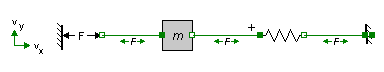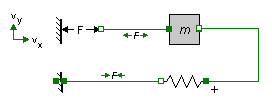﻿ 20-sim webhelp > Modeling Tutorial > Iconic Diagrams > Global Reference

# Global Reference

20-sim iconic diagram models do not contain geometrical information. It is not important how a model is drawn, but how the elements are connected. To make a correct interpretation of the simulation results, the definition of references has to be known.

## Across

Across variables in the top level of a model are always defined with respect to a single global reference. For all domains this global reference is equal to 0. The interpretation of this reference is up to the user. For example a zero pressure could be the absolute vacuum or the air pressure at ground level. For most physical domains, unless specified otherwise, a standard interpretation is used. This is shown in the table below.

 Domain Across (a) Global Reference power across a zero mechanical (translation) velocity v [m/s] zero velocity mechanical (rotation) angular velocity omega [rad/s] zero angular velocity pneumatic pressure p [Pa] air pressure at ground level thermal temperature T [K] absolute minimum temperature electric voltage u [V] zero voltage hydraulic pressure p [Pa] air pressure at ground level magnetic magnetomotoric force i [A] zero pseudothermal temperature T [K] absolute minimum temperature

## Through

Through variables in connections are always defined with respect to the components they are connected with.

### Non-Mechanical

Through variables that are not of the mechanical domain are always shown with an arrow indicating the direction of the positive flow.

### Mechanical

Through variables of the mechanical domain are always shown with two arrows indicating the direction of the positive force. Since mechanical variables are often geometrically interpreted, these arrows assume a positive velocity from the left to the right and from the bottom to the top.See for example the system shown above. Look at the connection between the spring and the fixed world. Most users will correctly interpret a positive force as one that "makes the mass slow down". The same model is shown below. To prevent an incorrect interpretation such as a positive force "makes the mass accelerate", the arrows are pointing inwards.This interpretation is only valid when you assume that a positive velocity is from the left to the right and from the bottom to the top!